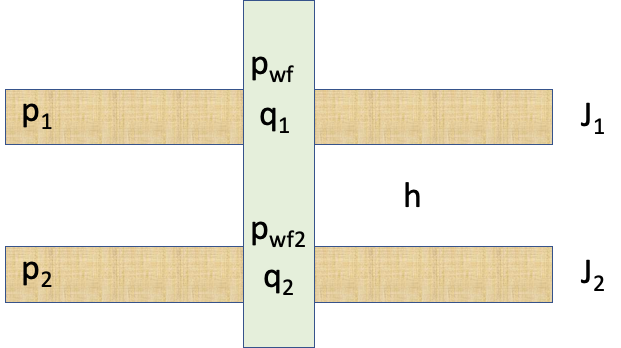# Multi-layer IPRFig. 1. Multi-layer well schematic

 (1)
 (2)
 (3)
 (4)

where

$//$ total subsurface flowrate of the well total well productivity Index apparent formation pressure of dual-layer formation bottom-hole pressure at the top of the reference layer $//$ bottom-hole pressure at the top of the $//$-th layer wellbore pressure loss between $//$-th layer and reference layer $//$ total subsurface flowrate of the  $//$-th layer formation pressure of the  $//$-th layer productivity Index of the  $//$-th layer

The above equations are valid for both producers $//0\end{array} //]]>$ and injectors $//$.

In many practical cases:

 (5)

where

$//$ wellbore fuid density gravity constant true vertical height between $//$-th layer and reference layer $//$